Function to create forest plots for objects of class "rma".

# S3 method for rma
xlim, alim, olim, ylim, at, steps=5,
text(c(-8.75,-5.25),     resk+3, c("Vaccinated", "Control"), cex=0.75, font=2) Additional summary estimates can be added to the plot with the addpoly function. See the documentation for that function for examples. When showweights=TRUE, the annotations will include information about the weights given to the observed outcomes during the model fitting. For simple models (such as those fitted with the rma.uni function), these weights correspond to the ‘inverse-variance weights’ (but are given in percent). For models fitted with the rma.mv function, the weights are based on the diagonal of the weight matrix. Note that the weighting structure is typically more complex in such models (i.e., the weight matrix is usually not just a diagonal matrix) and the weights shown therefore do not reflect this complexity. See weights for more details (for the special case that x is an intercept-only "rma.mv" model, one can also set showweights="rowsum" to show the ‘row-sum weights’). By default (i.e., when psize is not specified), the point sizes are a function of the square root of the model weights. This way, their areas are proportional to the weights. However, the point sizes are rescaled so that the smallest point size is plim and the largest point size is plim. As a result, their relative sizes (i.e., areas) no longer exactly correspond to their relative weights. If exactly relative point sizes are desired, one can set plim to NA, in which case the points are rescaled so that the smallest point size corresponds to plim and all other points are scaled accordingly. As a result, the largest point may be very large. Alternatively, one can set plim to NA, in which case the points are rescaled so that the largest point size corresponds to plim and all other points are scaled accordingly. As a result, the smallest point may be very small and essentially indistinguishable from the confidence interval line. To avoid the latter, one can also set plim, which enforces a minimal point size. With the shade argument, one can shade rows of the plot. The argument can be set to one of the following character strings: "zebra" (same as shade=TRUE) or "zebra2" to use zebra-style shading (starting either at the first or second study) or to "all" in which case all rows are shaded. Alternatively, the argument can be set to a logical vector to indicates for which studies the rows should be shaded. Finally, the argument can also be a numeric vector to indicate which rows to shade. The colshade argument can be used to set the color of shaded rows. ## Note The function tries to set some sensible values for the optional arguments, but it may be necessary to adjust these in certain circumstances. The function actually returns some information about the chosen values invisibly. Printing this information is useful as a starting point to make adjustments to the plot (see ‘Examples’). Arguments slab and ilab and when specifying vectors for arguments pch, psize, order, and/or colout (and when shade is a logical vector), the variables specified are assumed to be of the same length as the data originally passed to the model fitting function (and if the data argument was used in the original model fit, then the variables will be searched for within this data frame first). Any subsetting and removal of studies with missing values is automatically applied to the variables specified via these arguments. If the number of studies is quite large, the labels, annotations, and symbols may become quite small and impossible to read. Stretching the plot window vertically may then provide a more readable figure (one should call the function again after adjusting the window size, so that the label/symbol sizes can be properly adjusted). Also, the cex, cex.lab, and cex.axis arguments are then useful to adjust the symbol and text sizes. If the outcome measure used for creating the plot is bounded (e.g., correlations are bounded between -1 and +1, proportions are bounded between 0 and 1), one can use the olim argument to enforce those limits (the observed outcomes and confidence/prediction intervals cannot exceed those bounds then). The models without moderators, the col argument can also be a vector of two elements, the first for specifying the color of the summary polygon, the second for specifying the color of the line for the prediction interval. The lty argument can also be a vector of up to three elements, the first for specifying the line type of the individual CIs ("solid" by default), the second for the line type of the prediction interval ("dotted" by default), the third for the line type of the horizontal lines that are automatically added to the plot ("solid" by default; set to "blank" to remove them). ## Additional Arguments There are some additional arguments that can be passed to the function via ... (hence, they cannot be abbreviated): top the amount of space to leave empty at the top of the plot (e.g., for adding headers) (the default is 3 rows). annosym optional vector of length 3 to select the left bracket, separation, and right bracket symbols for the annotations (the default is c(" [", ", ", "]")). Can also include a 4th element to adjust the look of the minus symbol, for example to use a proper minus (−) sign instead of a hyphen-minus (-). textpos optional vector of length 2 to specify the placement of the study labels and the annotations (the default is to use the horizontal limits of the plot region, i.e., the study labels to the right of xlim and the annotations to the left of xlim). rowadj optional vector of length 3 to vertically adjust the position of the study labels, the annotations, and the extra information (if specified via ilab). This is useful for fine-tuning the position of text added with different positional alignments (i.e., argument pos in the text function). ## Author Wolfgang Viechtbauer wvb@metafor-project.org https://www.metafor-project.org ## See also forest for an overview of the various forest functions and forest.default for the function draw forest plots without a summary polygon. rma.uni, rma.mh, rma.peto, rma.glmm, and rma.mv for functions to fit models for which forest plots can be drawn. addpoly for a function to add polygons to forest plots. ## Examples ### meta-analysis of the log risk ratios using a random-effects model res <- rma(measure="RR", ai=tpos, bi=tneg, ci=cpos, di=cneg, data=dat.bcg, slab=paste(author, year, sep=", ")) ### default forest plot of the log risk ratios and summary estimate forest(res, header=TRUE) ### summary estimate in row -1; studies in rows k=13 through 1; horizontal ### lines in rows 0 and k+1; two extra lines of space at the top for headings, ### and other annotations; headings (if requested) in line k+2 op <- par(xpd=TRUE) text(x=-8.1, y=-1:16, -1:16, pos=4, cex=0.6, col="red")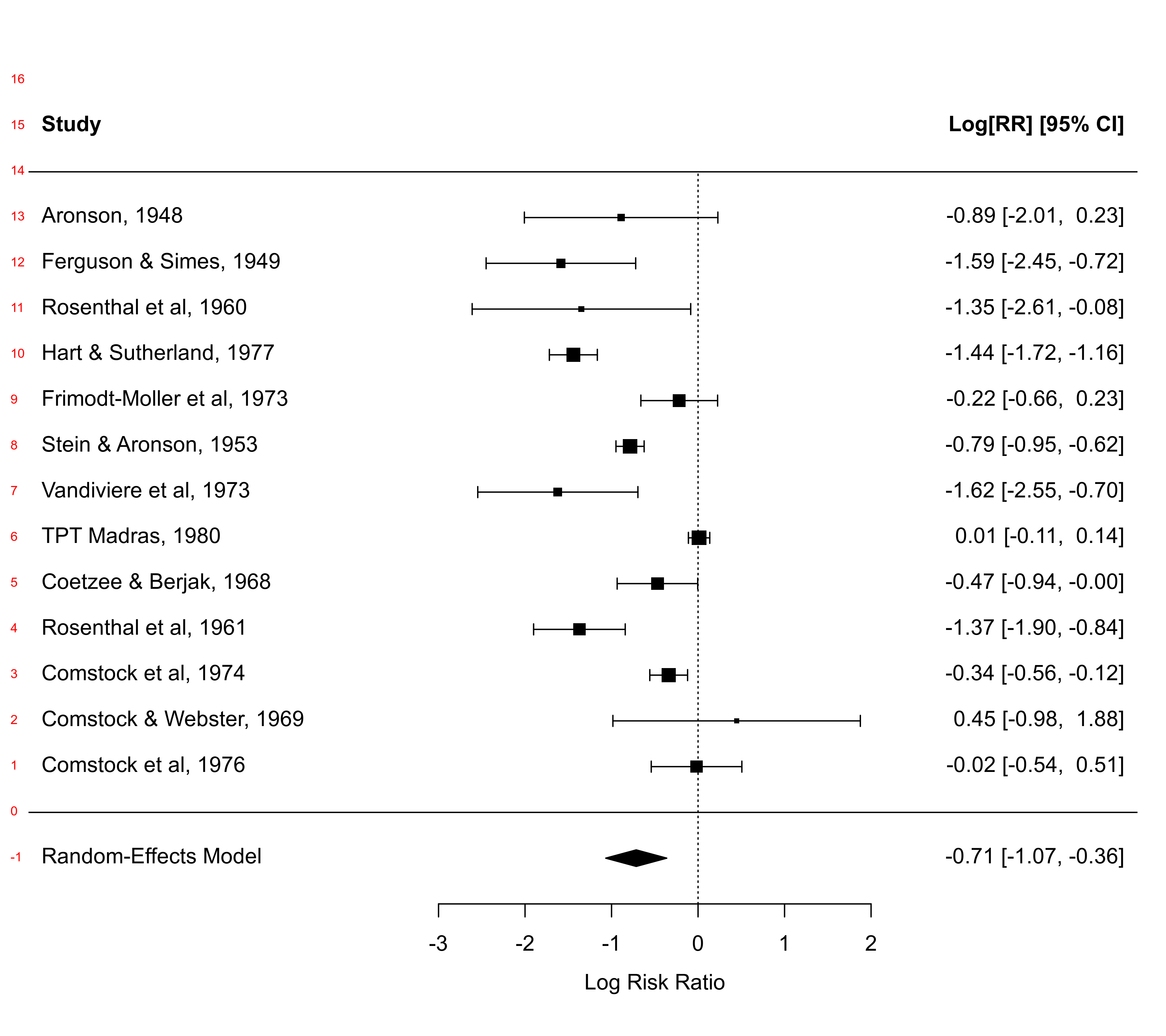par(op) ### can also inspect defaults chosen defaults <- forest(res)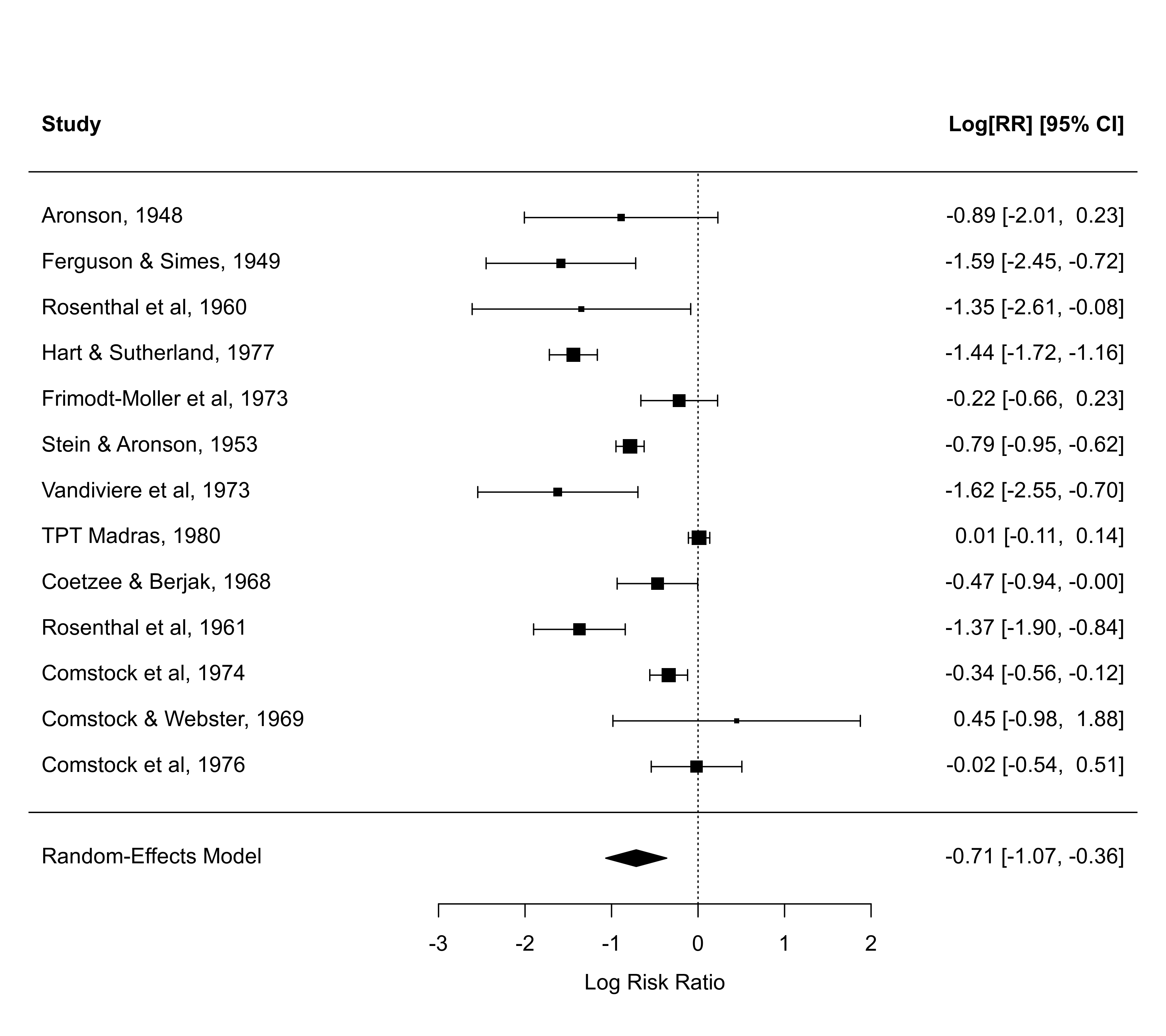defaults #>xlim
#>  -7.74  5.08
#>
#> $alim #>  -3 2 #> #>$at
#>  -3 -2 -1  0  1  2
#>
#> $ylim #>  -1.5 16.0 #> #>$rows
#>    1  2  3  4  5  6  7  8  9 10 11 12 13
#>
#> $cex #>  1 #> #>$cex.lab
#>  1
#>
#> $cex.axis #>  1 #> #>$ilab.xpos
#> NULL
#>
#> $ilab.pos #> NULL #> #>$textpos
#>  -7.74  5.08
#>
#> $areas #>  0.40 0.35 0.25 #> ### several forest plots illustrating the use of various arguments forest(res, cex=0.8)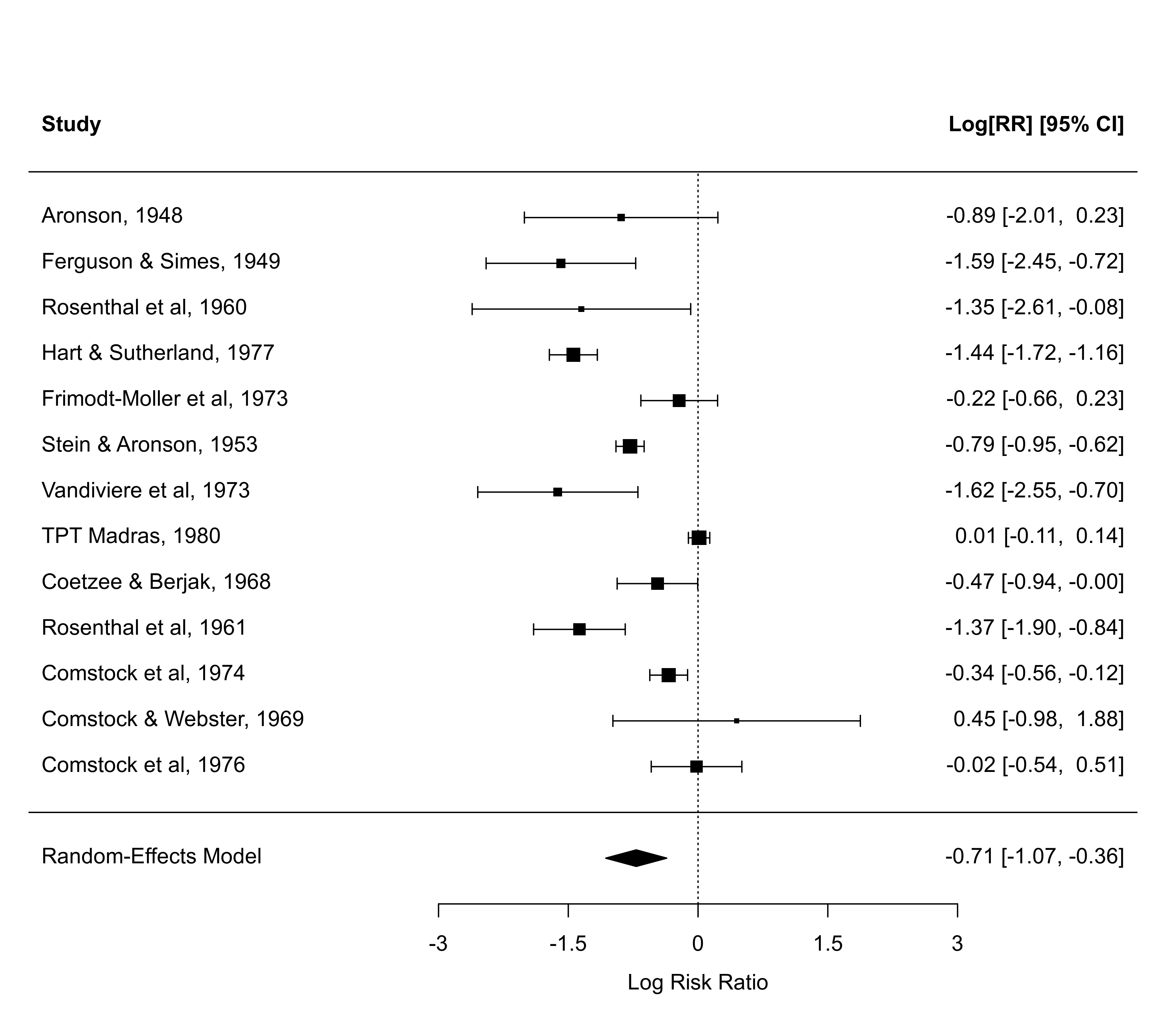forest(res, cex=0.8, addpred=TRUE)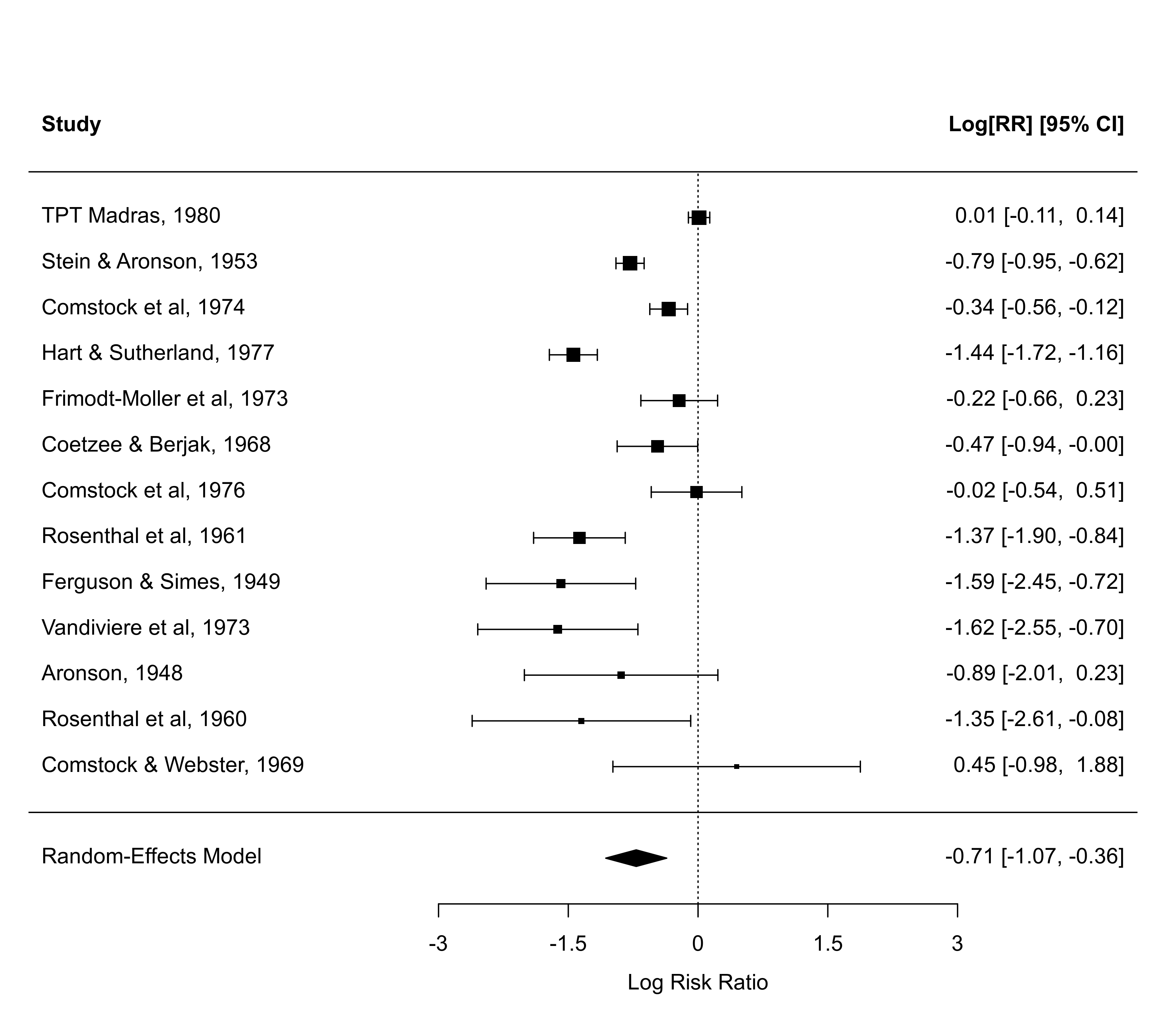forest(res, cex=0.8, alim=c(-3,3))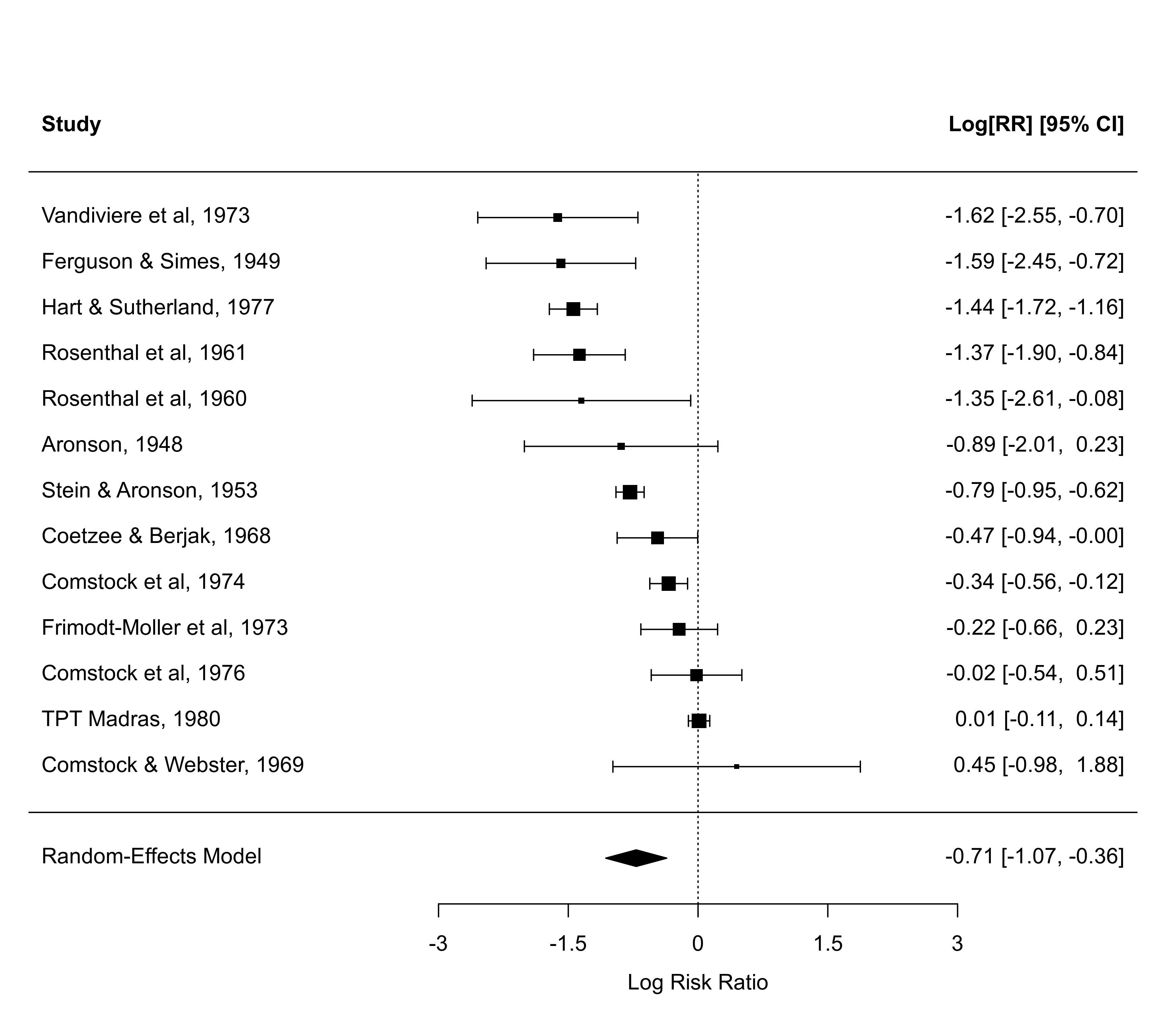forest(res, cex=0.8, alim=c(-3,3), order="prec")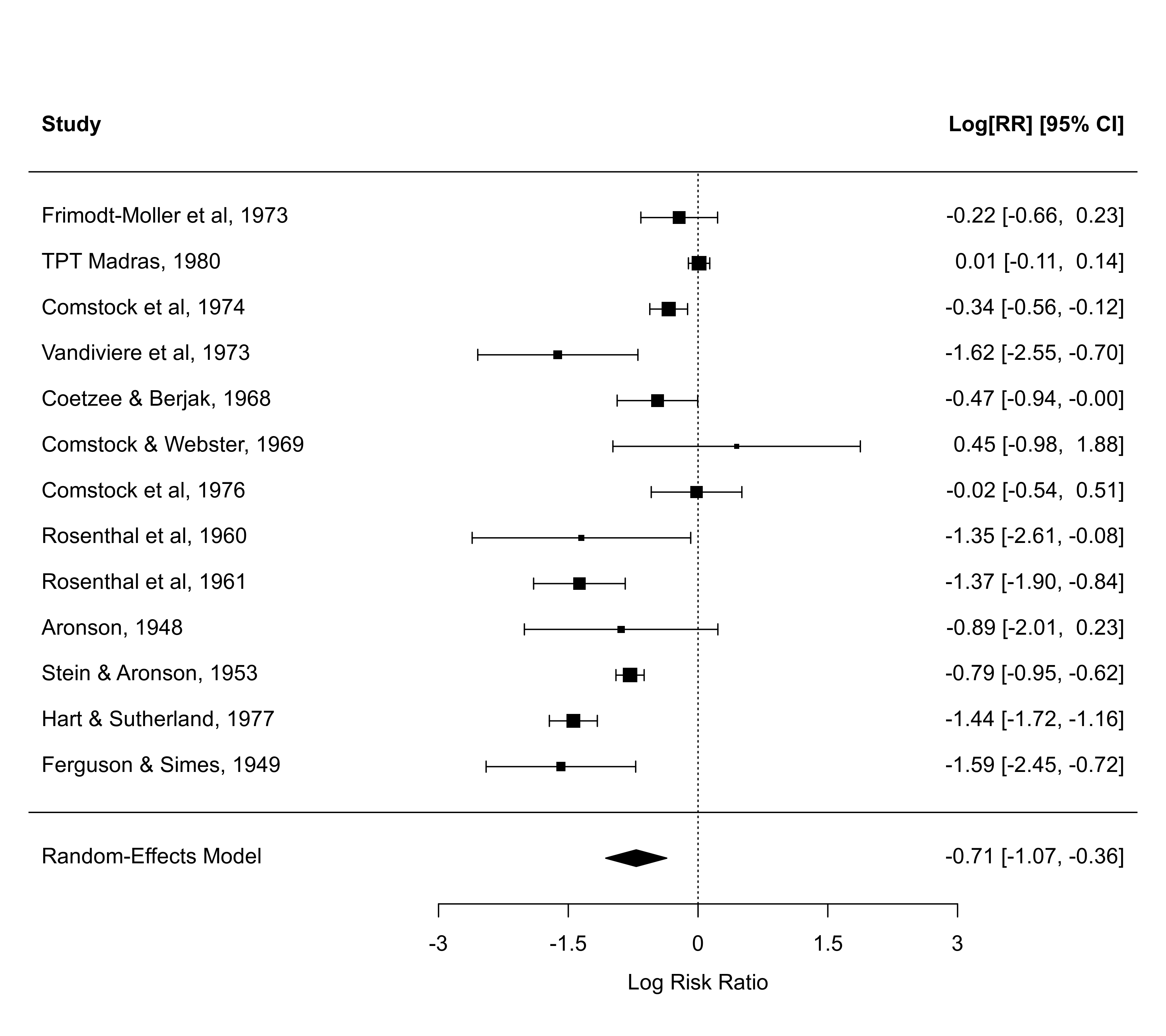forest(res, cex=0.8, alim=c(-3,3), order="obs")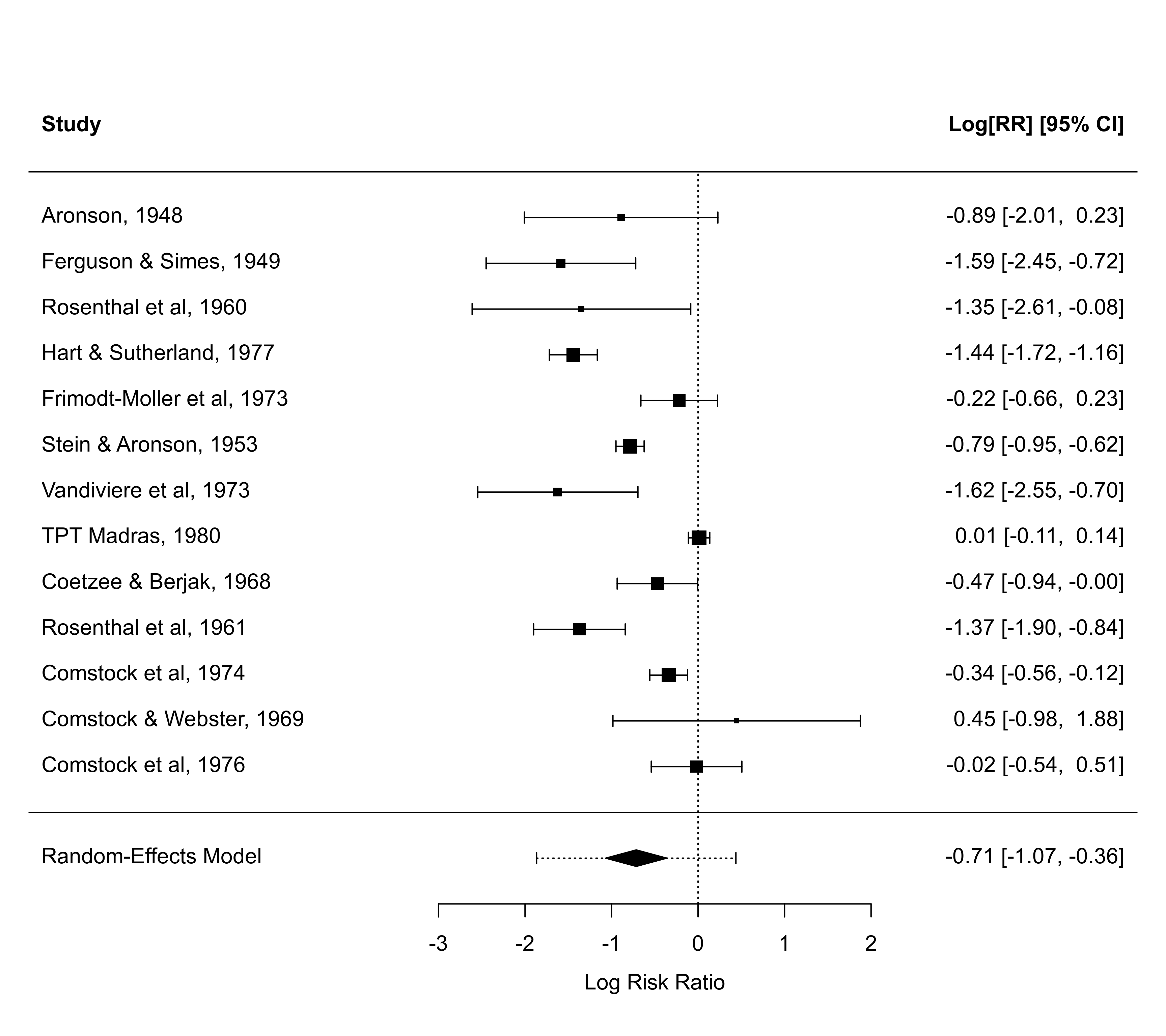forest(res, cex=0.8, alim=c(-3,3), order=ablat)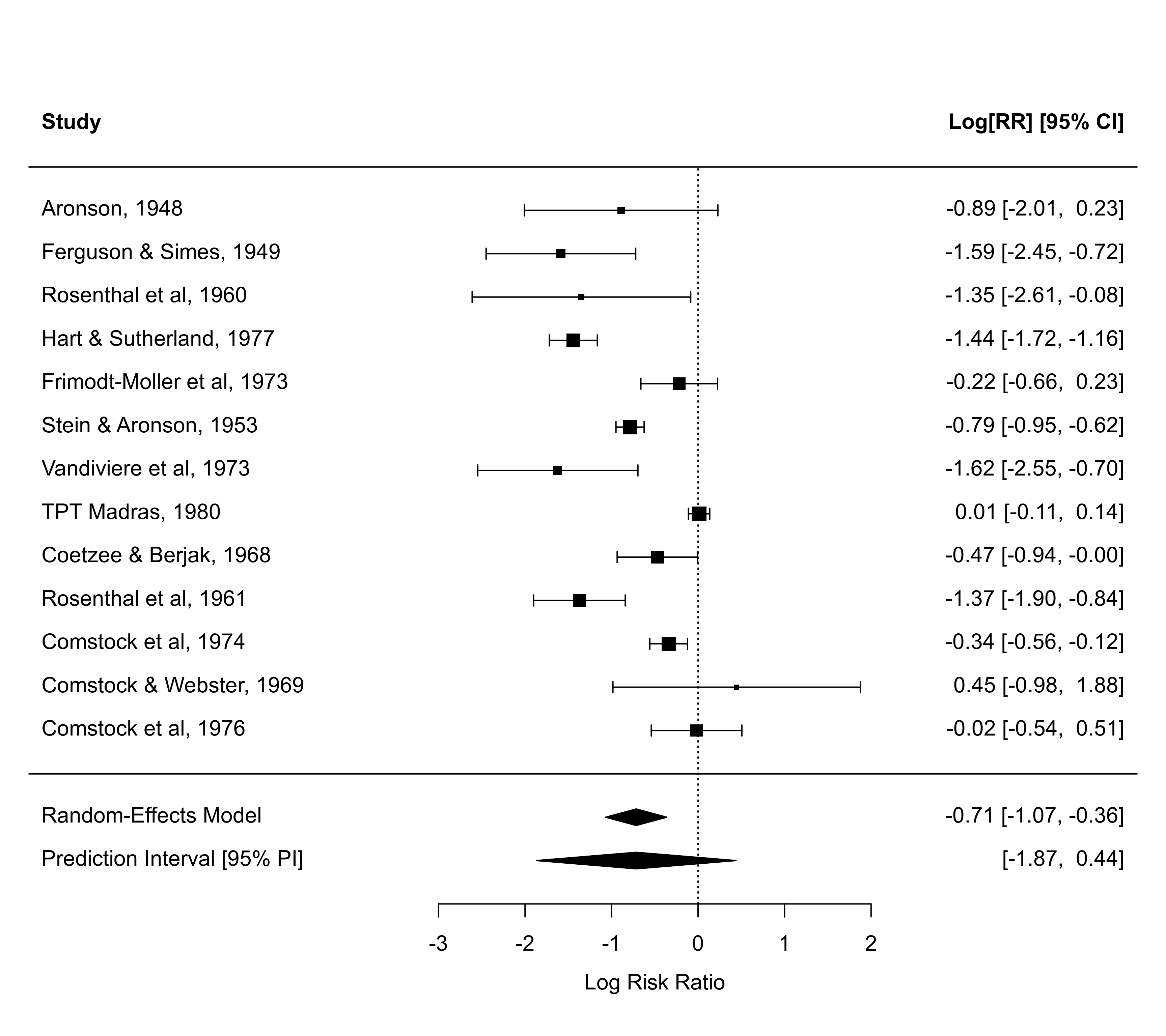### adjust xlim values to see how that changes the plot forest(res)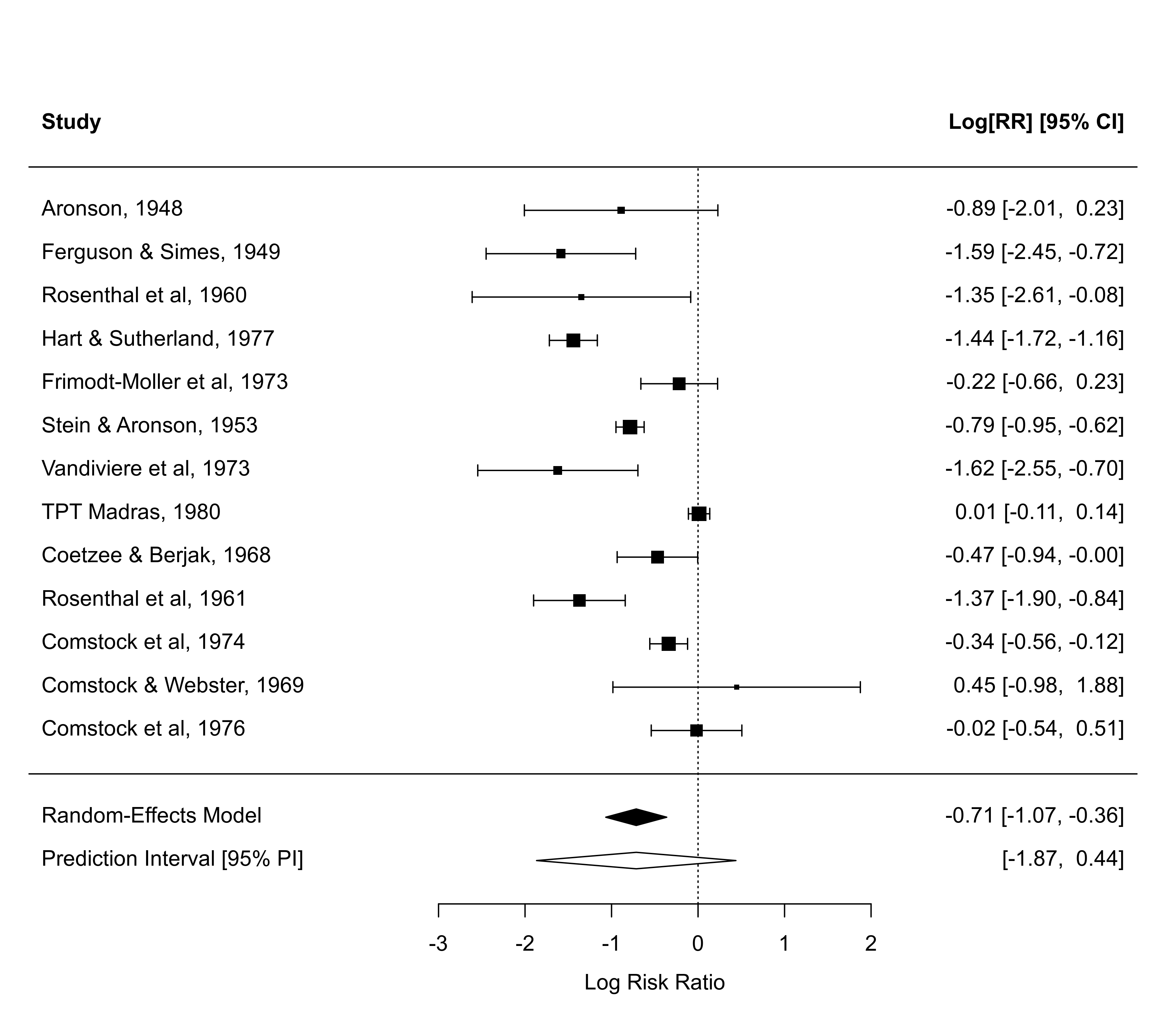par("usr")[1:2] # this shows what xlim values were chosen by default #>  -7.74 5.08 forest(res, xlim=c(-16,14))forest(res, xlim=c(-18,10))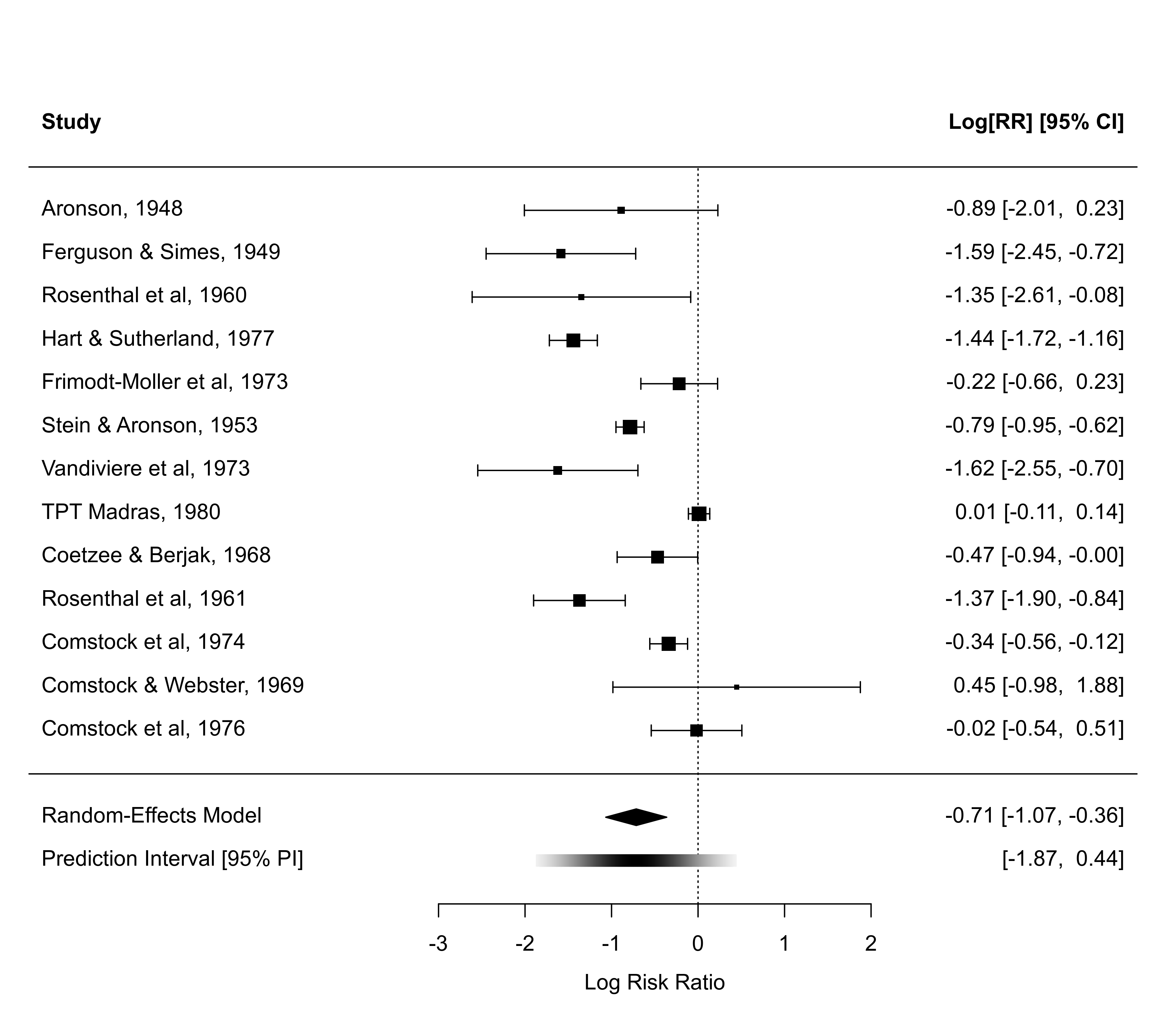forest(res, xlim=c(-10,12))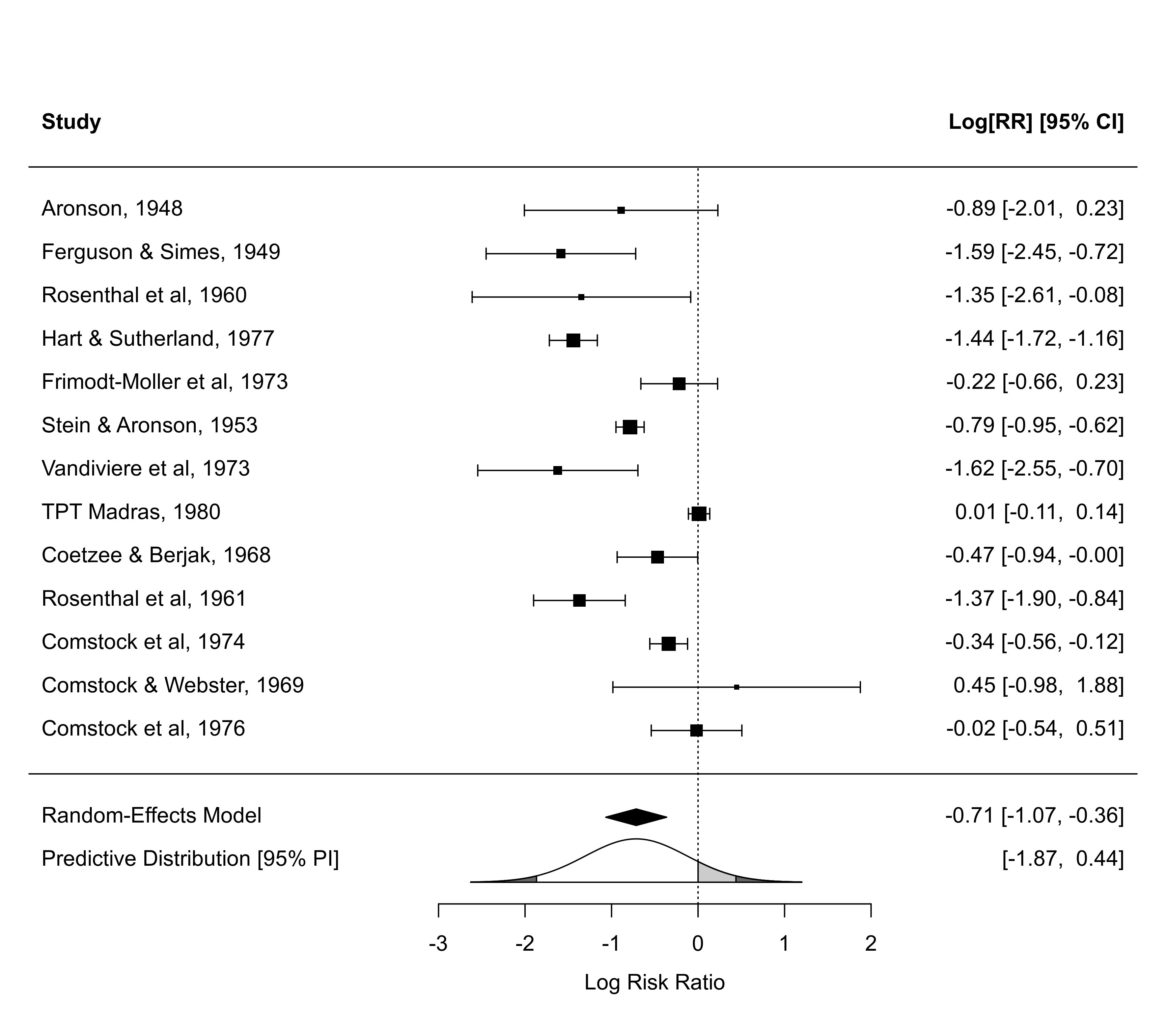### illustrate transf argument forest(res, transf=exp, at=0:7, xlim=c(-8,12), cex=0.8, refline=1, header=TRUE)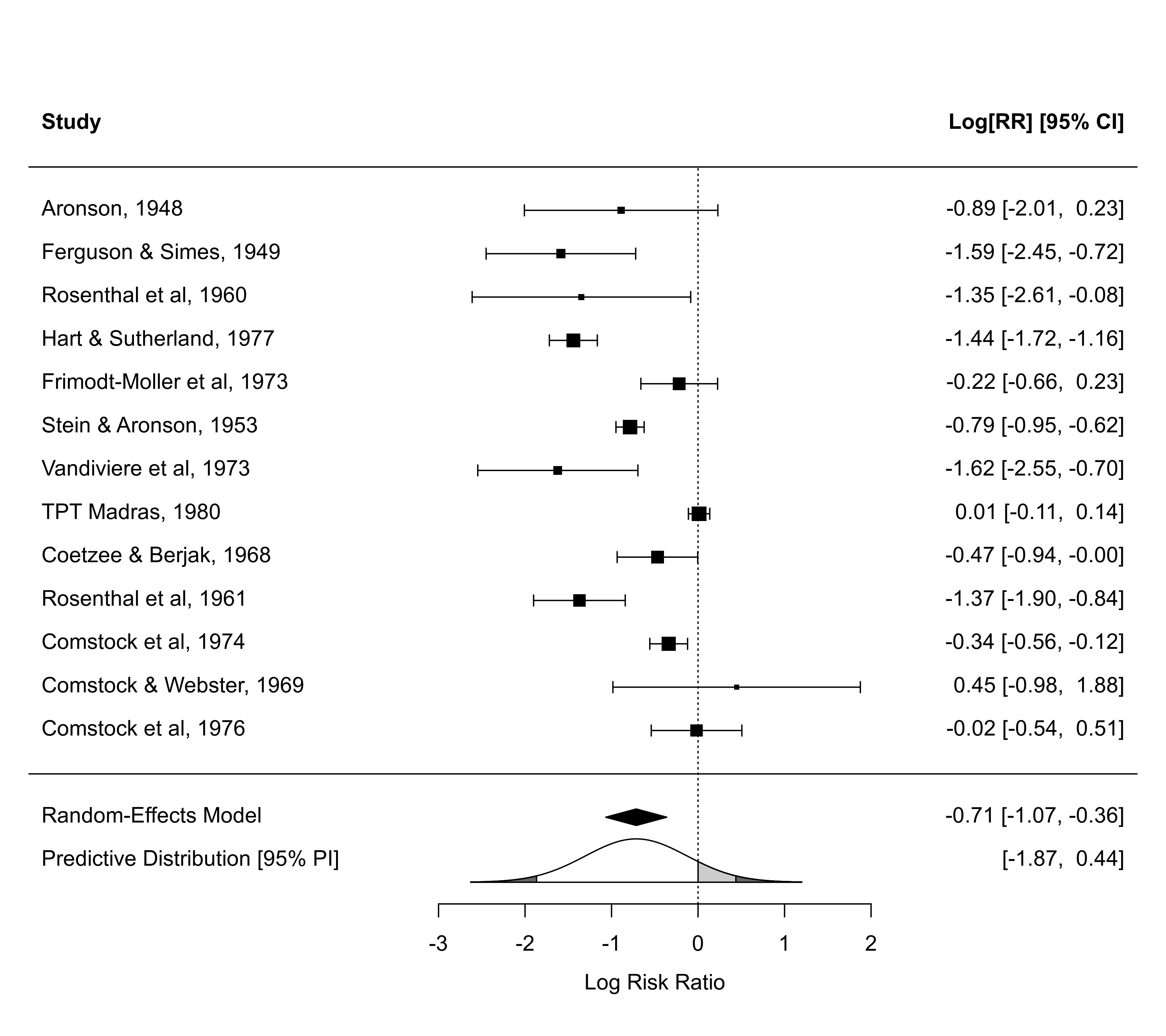### illustrate atransf argument forest(res, atransf=exp, at=log(c(0.05,0.25,1,4,20)), xlim=c(-8,7), cex=0.8, header=TRUE)### showweights argument forest(res, atransf=exp, at=log(c(0.05,0.25,1,4,20)), xlim=c(-8,8), order="prec", showweights=TRUE, cex=0.8)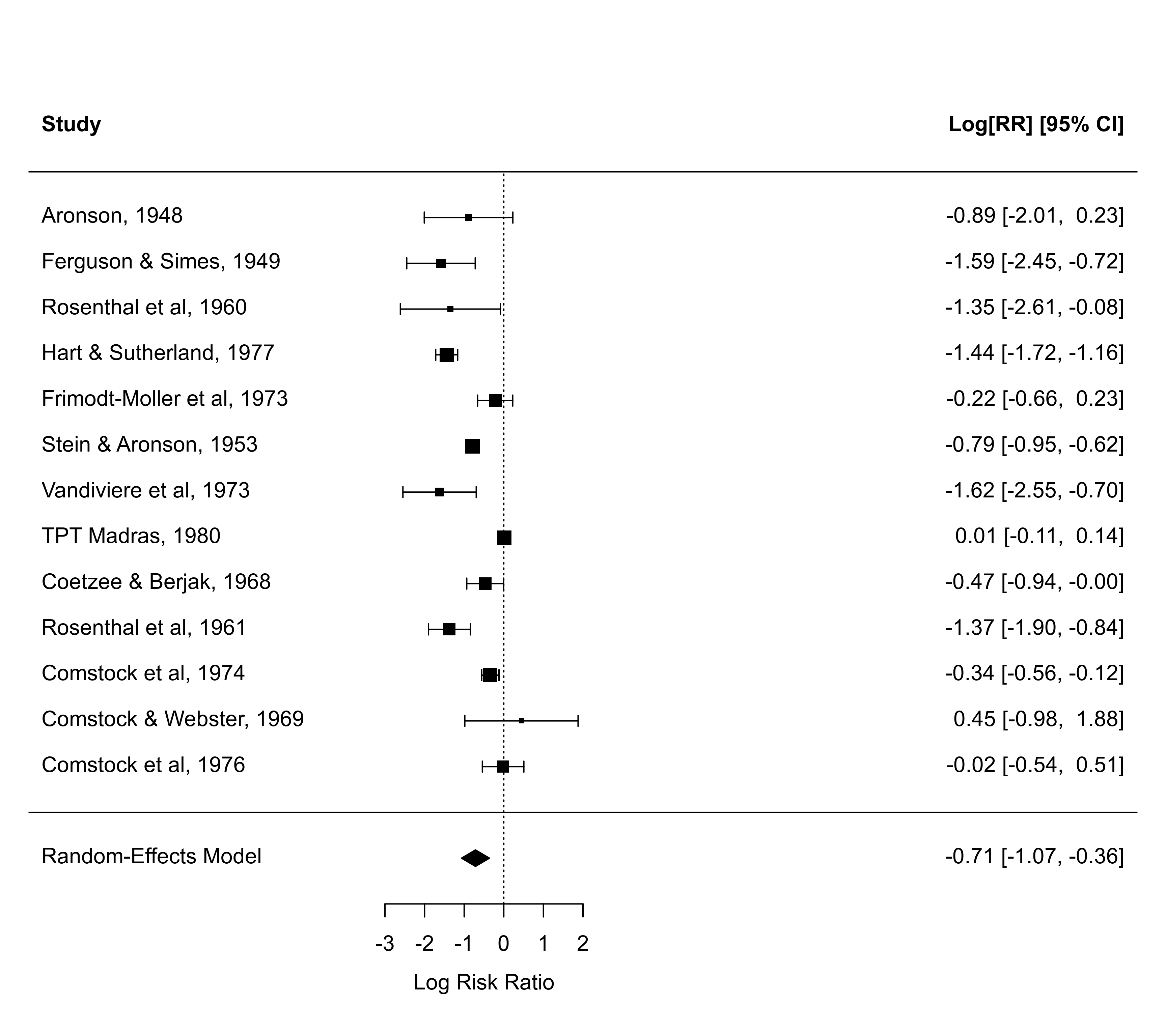### illustrade shade argument forest(res, header=TRUE, shade="zebra")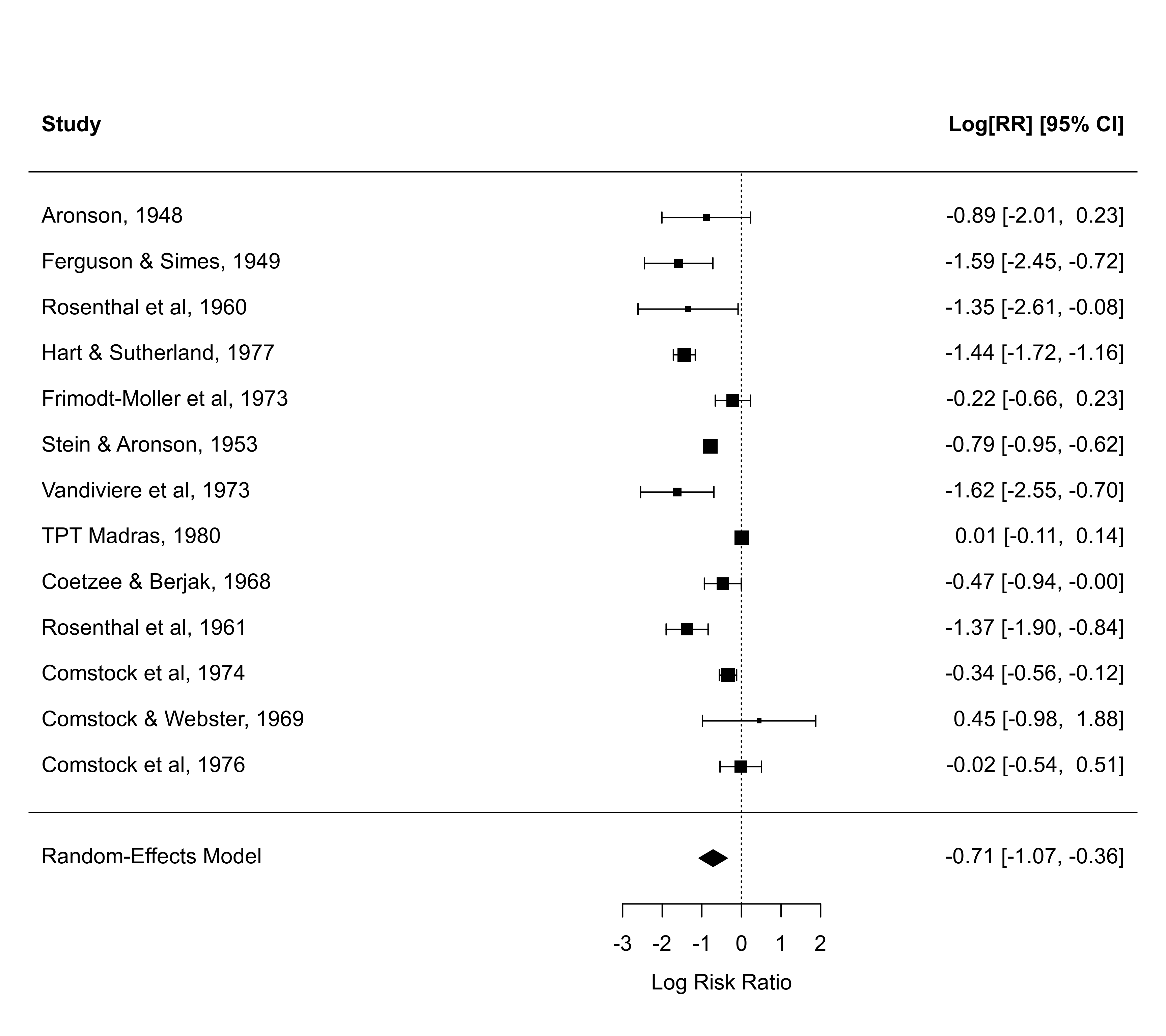forest(res, header=TRUE, shade=year >= 1970)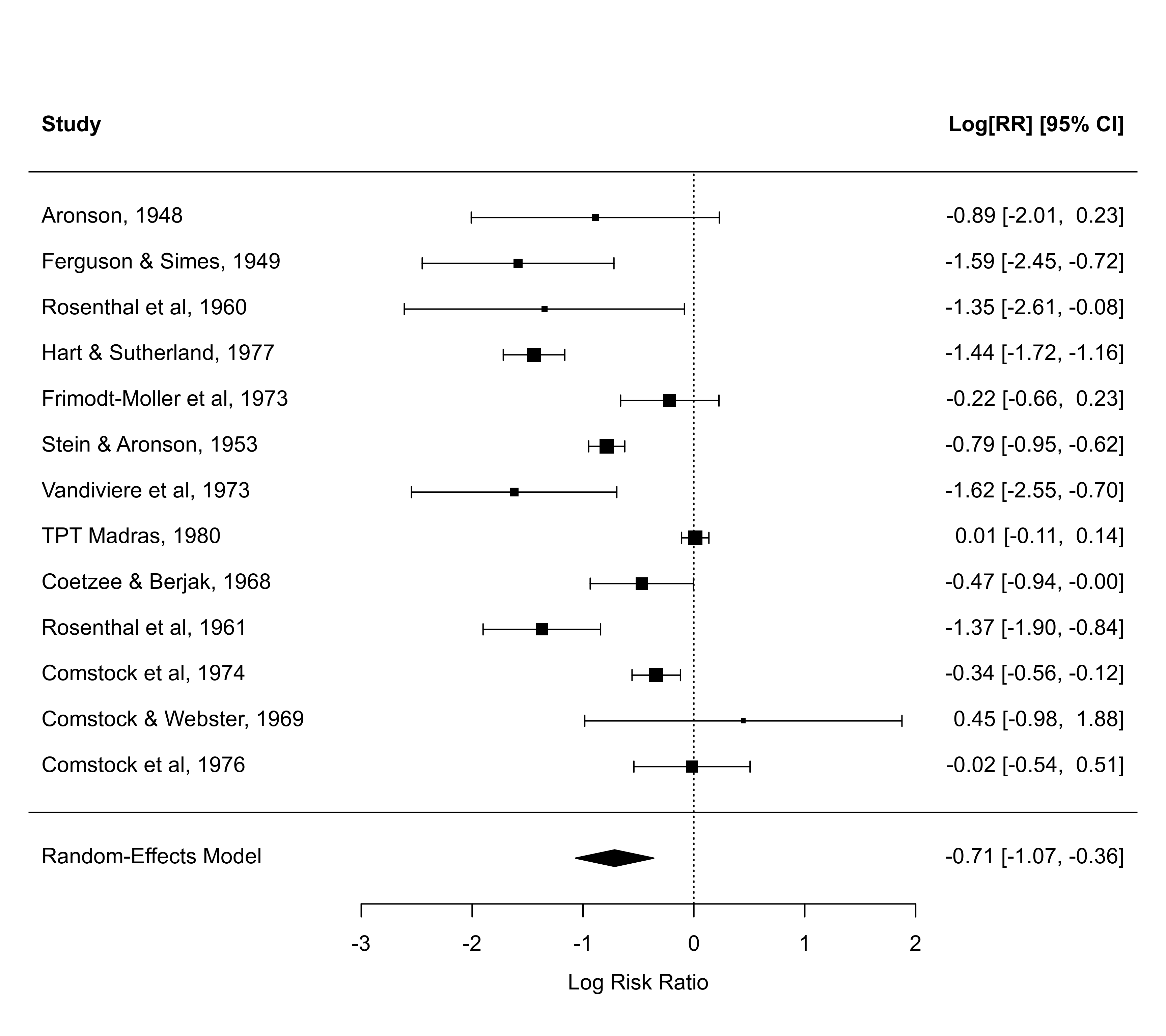forest(res, header=TRUE, shade=c(1,5,10))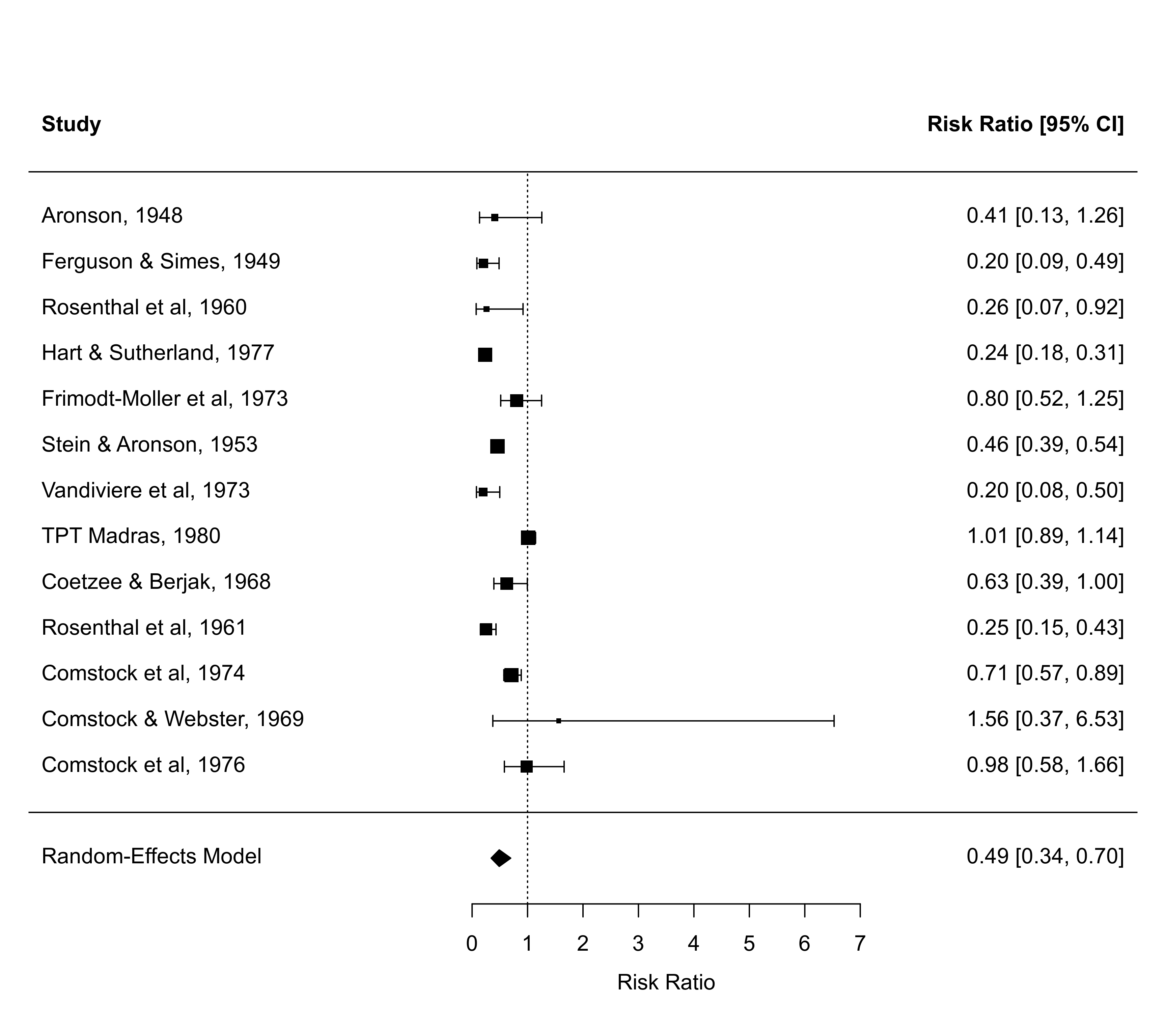### forest plot with extra annotations ### note: may need to widen plotting device to avoid overlapping text forest(res, atransf=exp, at=log(c(0.05, 0.25, 1, 4)), xlim=c(-16,6), ilab=cbind(tpos, tneg, cpos, cneg), ilab.xpos=c(-9.5,-8,-6,-4.5), cex=0.75, header="Author(s) and Year") op <- par(cex=0.75, font=2) text(c(-9.5,-8,-6,-4.5), res$k+2, c("TB+", "TB-", "TB+", "TB-"))
text(c(-8.75,-5.25),     res$k+3, c("Vaccinated", "Control"))par(op) ### mixed-effects model with absolute latitude as moderator res <- rma(measure="RR", ai=tpos, bi=tneg, ci=cpos, di=cneg, mods = ~ ablat, data=dat.bcg, slab=paste(author, year, sep=", ")) ### forest plot with observed and fitted values forest(res, xlim=c(-9,5), order="fit", cex=0.8, ilab=ablat, ilab.xpos=-4, atransf=exp, at=log(c(0.05,0.25,1,4)), header="Author(s) and Year") text(-4, res$k+2, "Latitude", cex=0.8, font=2)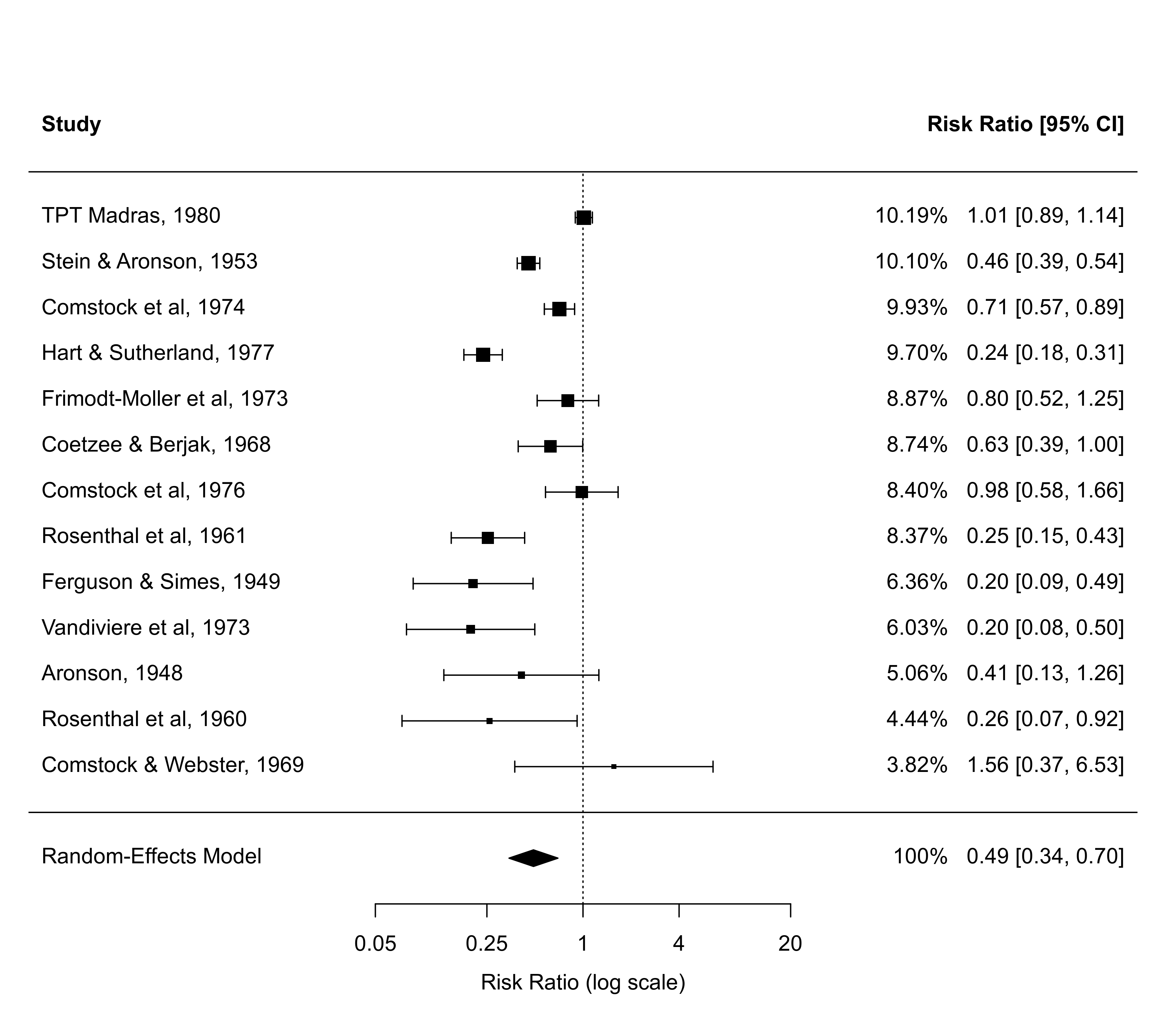### meta-analysis of the log risk ratios using a random-effects model
res <- rma(measure="RR", ai=tpos, bi=tneg, ci=cpos, di=cneg, data=dat.bcg,
slab=paste(author, year, sep=", "))

### for more complicated plots, the ylim and rows arguments may be useful
forest(res)
forest(res, ylim=c(-1.5, 16)) # the default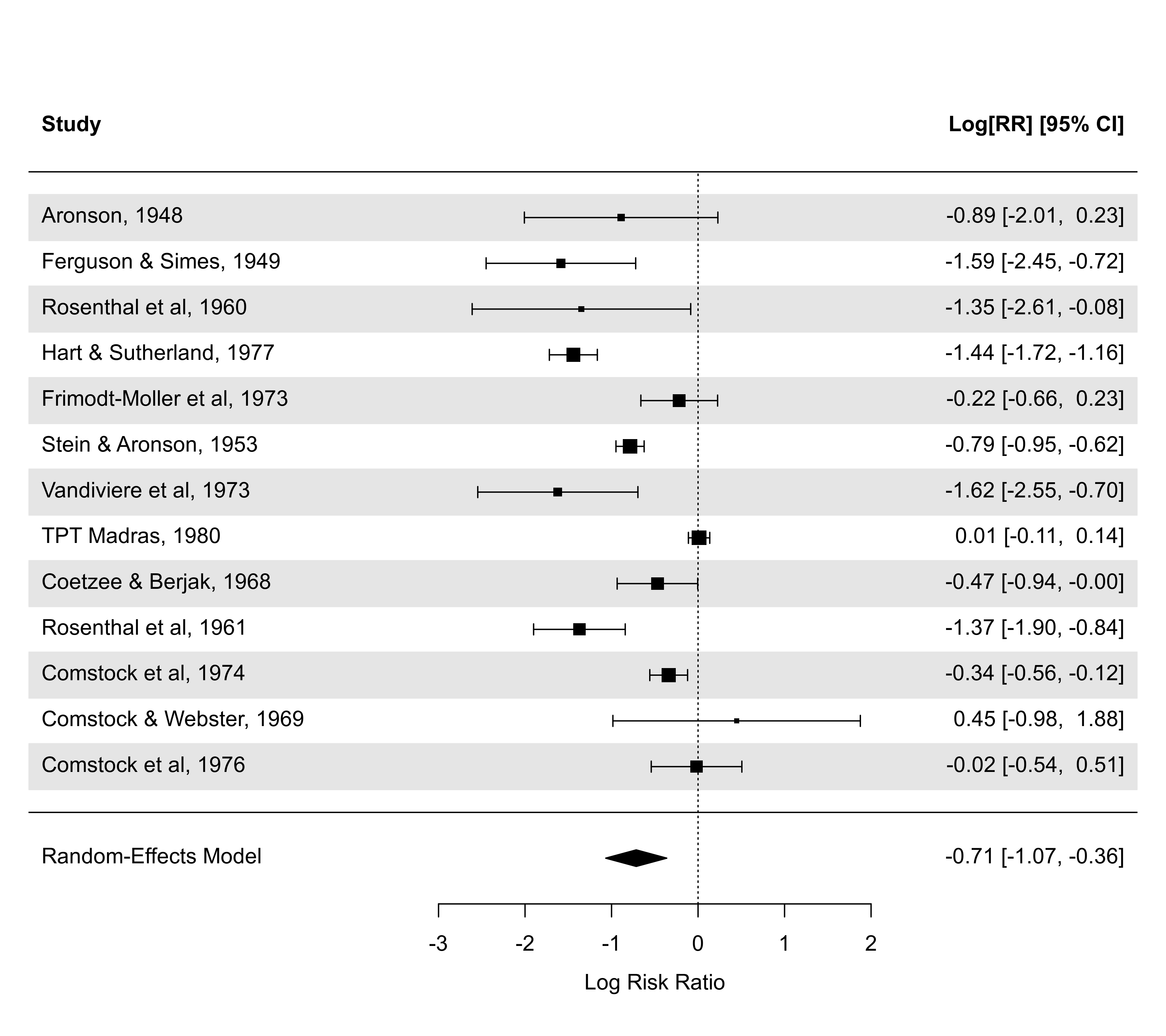forest(res, ylim=c(-1.5, 20)) # extra space in plot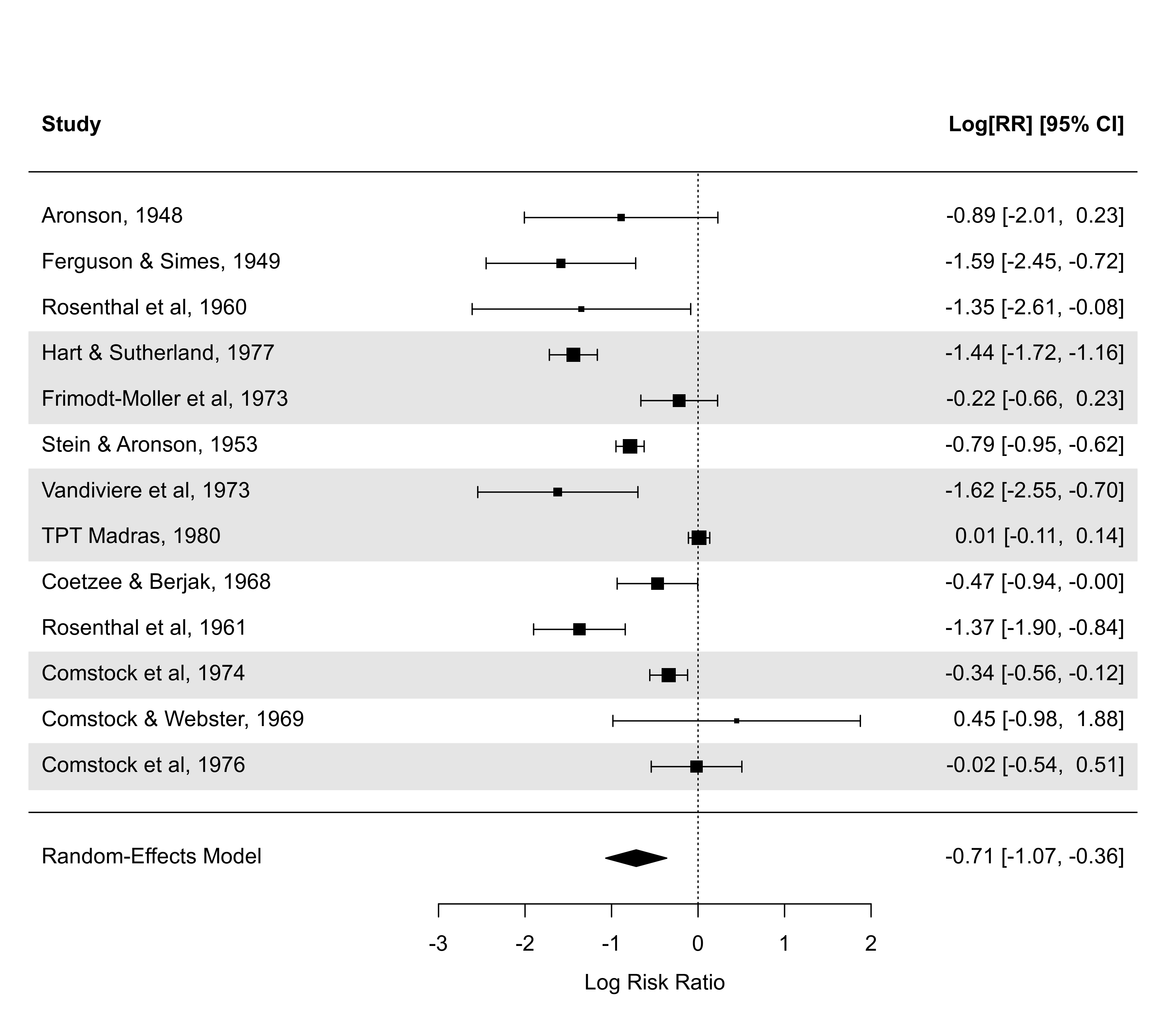forest(res, ylim=c(-1.5, 20), rows=c(17:15, 12:6, 3:1)) # set positions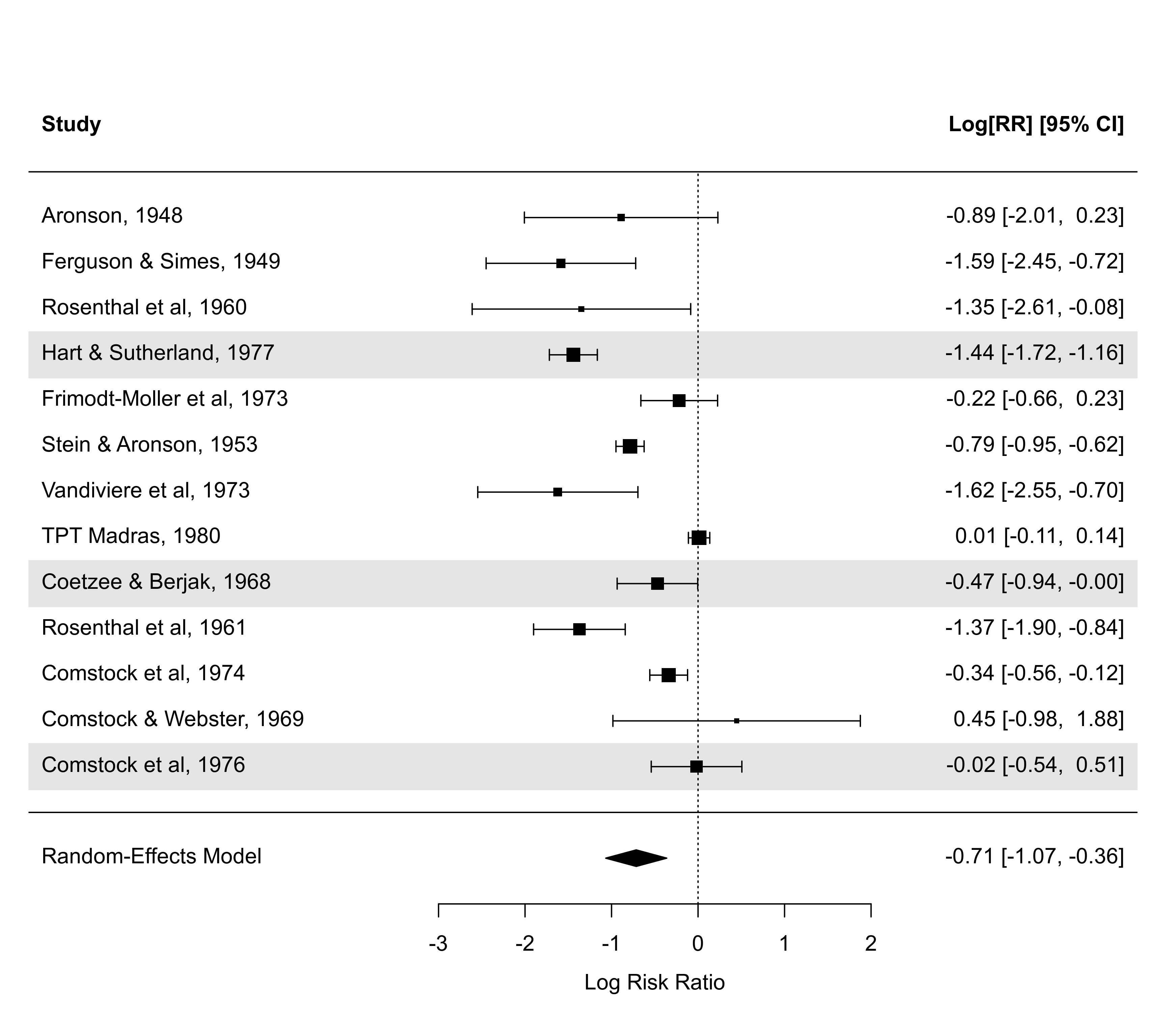### forest plot with subgrouping of studies
### note: may need to widen plotting device to avoid overlapping text
tmp <- forest(res, xlim=c(-16, 4.6), at=log(c(0.05, 0.25, 1, 4)), atransf=exp,
ilab=cbind(tpos, tneg, cpos, cneg), ilab.xpos=c(-9.5,-8,-6,-4.5),
cex=0.75, ylim=c(0.5, 21),
order=alloc, rows=c(1:2,5:11,14:17),
op <- par(cex=0.75, font=2)
text(c(-9.5,-8,-6,-4.5), tmp$ylim-1, c("TB+", "TB-", "TB+", "TB-")) text(c(-8.75,-5.25), tmp$ylim,   c("Vaccinated", "Control"))
par(font=4)
text(-16, c(18,12,3), c("Systematic Allocation", "Random Allocation",
"Alternate Allocation"), pos=4)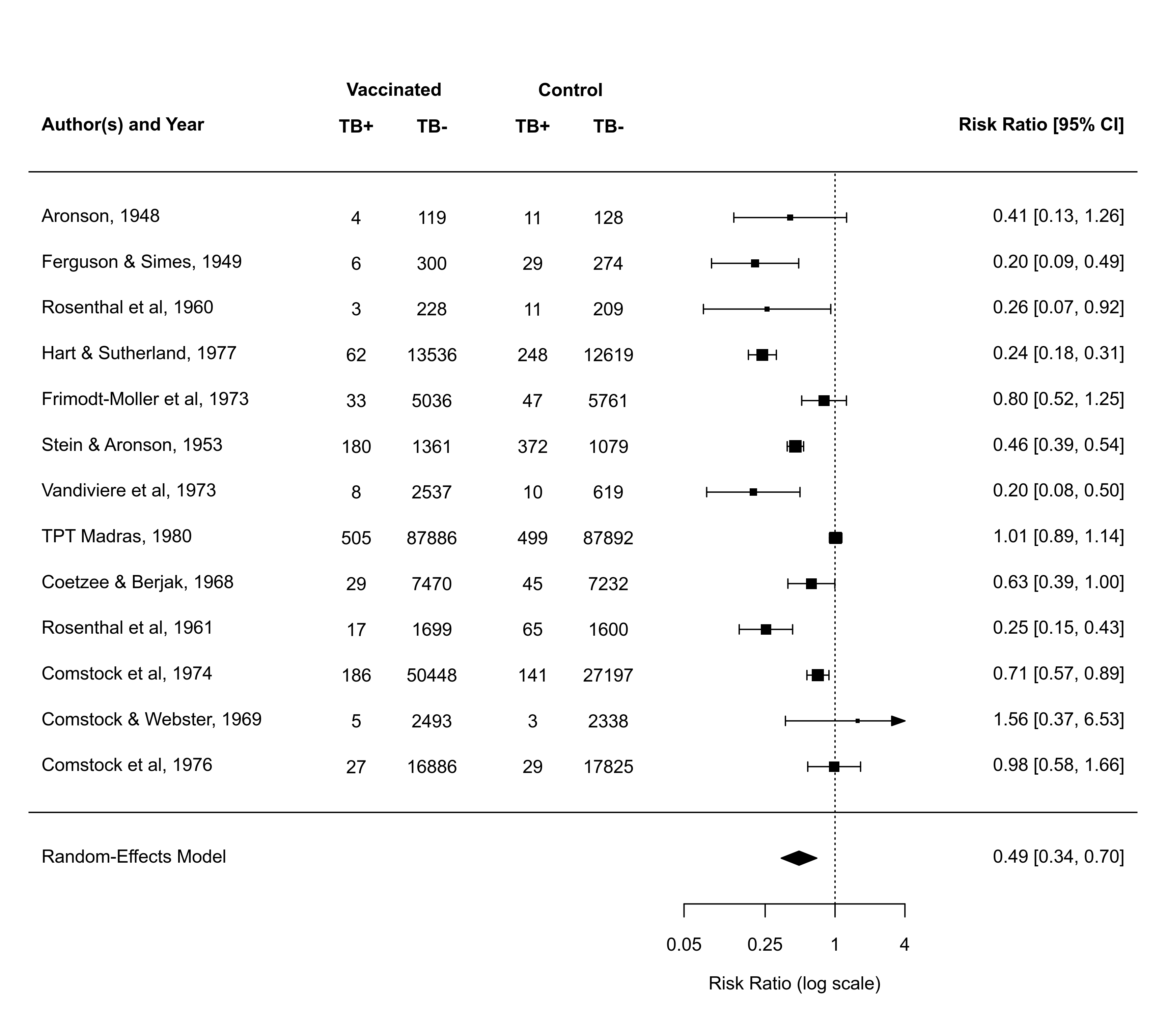par(op)

### for the three subgroups are added to such a forest plot

### illustrate use of olim argument with a meta-analysis of raw correlation
### coefficients (data from Pritz, 1997); without olim=c(0,1), some of the
### CIs would have upper bounds larger than 1
dat <- escalc(measure="PR", xi=xi, ni=ni, data=dat.pritz1997)
res <- rma(yi, vi, data=dat, slab=paste0(study, ") ", authors))
forest(res, xlim=c(-0.8,1.6), alim=c(0,1), psize=1, refline=coef(res), olim=c(0,1), header=TRUE)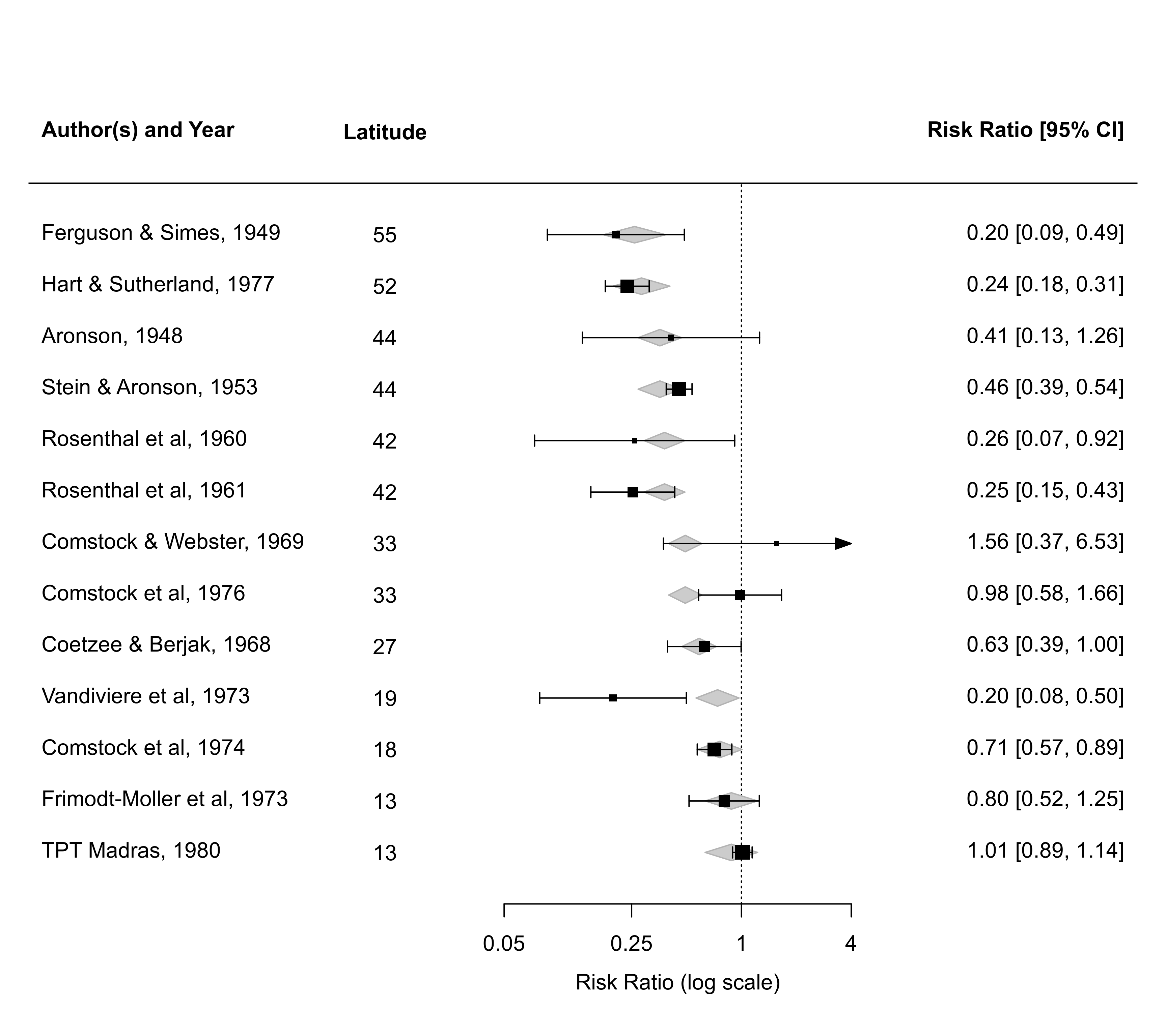### an example of a forest plot where the data have a multilevel structure and
### we want to reflect this by grouping together estimates from the same cluster
dat <- dat.konstantopoulos2011
res <- rma.mv(yi, vi, random = ~ 1 | district/school, data=dat,
slab=paste0("District ", district, ", School: ", school))
dd <- c(0,diff(dat$district)) dd[dd > 0] <- 1 rows <- (1:res$k) + cumsum(dd)
op <- par(tck=-0.01, mgp = c(1.6,0.2,0), mar=c(3,8,1,6))